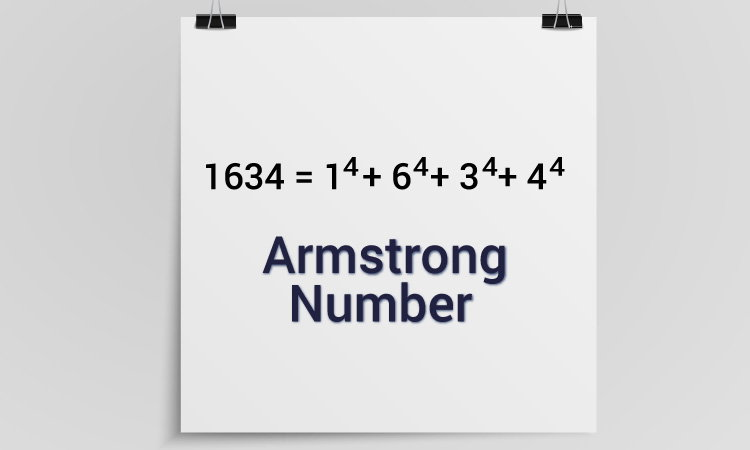# C Program to Check Armstrong Number

In this example, you will learn to check whether an integer entered by the user is an Armstrong number or not.To understand this example, you should have the knowledge of the following C programming topics:

A positive integer is called an Armstrong number (of order n) if

``````abcd... = an + bn + cn + dn + �
``````

In the case of an Armstrong number of 3 digits, the sum of cubes of each digit is equal to the number itself. For example, 153 is an Armstrong number because

```153 = 1*1*1 + 5*5*5 + 3*3*3
```

## Check Armstrong Number of three digits

``````#include <stdio.h>
int main() {
int num, originalNum, remainder, result = 0;
printf("Enter a three-digit integer: ");
scanf("%d", &num);
originalNum = num;

while (originalNum != 0) {
remainder = originalNum % 10;
result += remainder * remainder * remainder;
originalNum /= 10;
}

if (result == num)
printf("%d is an Armstrong number.", num);
else
printf("%d is not an Armstrong number.", num);

return 0;
}

``````

Output

```Enter a three-digit integer: 371
371 is an Armstrong number.
```

## Check Armstrong Number of n digits

``````#include <math.h>
#include <stdio.h>

int main() {
int num, originalNum, remainder, n = 0;
float result = 0.0;
// printf("Enter an integer: ");
// scanf("%d", &num);

num = 153;
originalNum = num;

while (originalNum != 0) {
originalNum /= 10;
++n;
}
originalNum = num;

while (originalNum != 0) {
remainder = originalNum % 10;
result += pow(remainder, n);
originalNum /= 10;
}

if ((int)result == num)
printf("%d is an Armstrong number.", num);
else
printf("%d is not an Armstrong number.", num);
return 0;
}
``````

Output

```Enter an integer: 1634
1634 is an Armstrong number.
```

In this program, the number of digits of an integer is calculated first and stored in `n`. And, the `pow()` function is used to compute the power of individual digits in each iteration of the `while` loop.